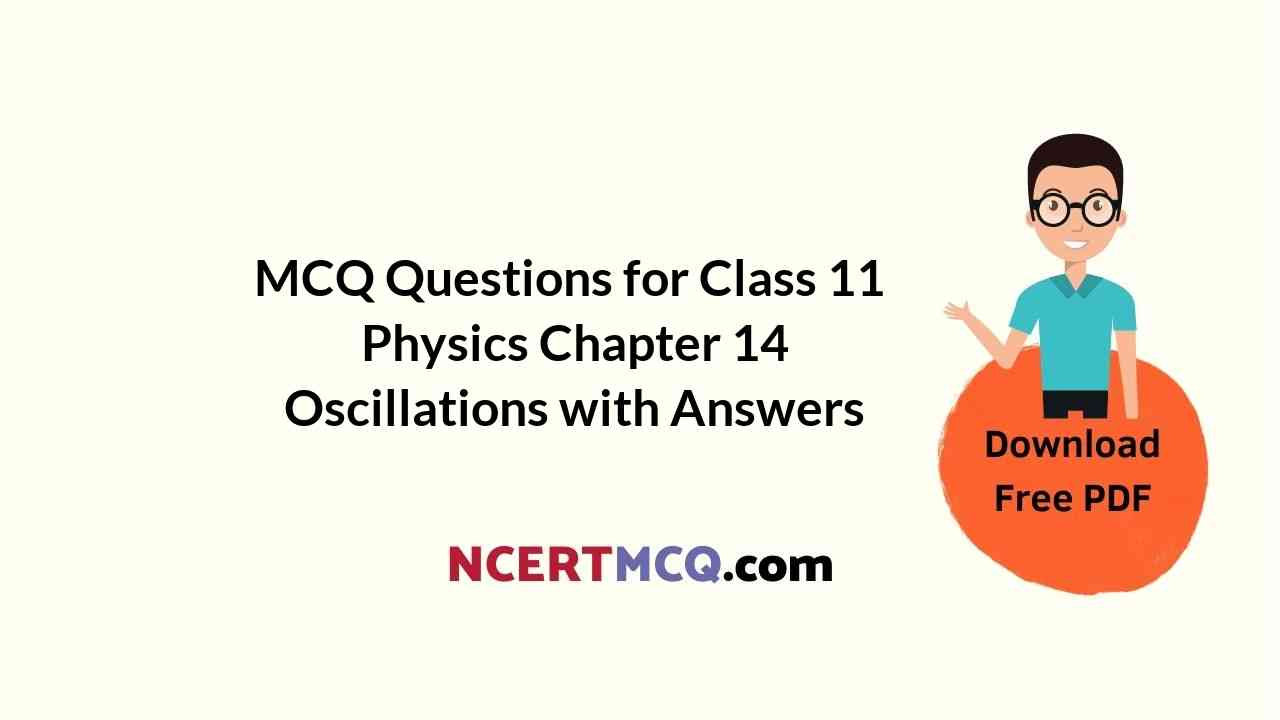Check the below NCERT MCQ Questions for Class 11 Physics Chapter 14 Oscillations with Answers Pdf free download. MCQ Questions for Class 11 Physics with Answers were prepared based on the latest exam pattern. We have provided Oscillations Class 11 Physics MCQs Questions with Answers to help students understand the concept very well.

## Oscillations Class 11 MCQs Questions with Answers

MCQ On Oscillations Class 11 Chapter 14 Question 1.
In damped oscilation the directions of the restoring force and the resistive force
(a) are the same
(b) are opposite
(c) may be same or opposite
(d) have no relation with each other

Answer: (c) may be same or opposite

Oscillation MCQ With Solution Pdf Chapter 14 Class 11  Question 2.
The displacement y of a particle executing periodic motion is given by y = 4 cos² (t/2) sin (1000t). This expression may be considered to be a result of superposition of the following number of harmonic motions.
(a) two
(b) three
(c) four
(d) five

Oscillation MCQ With Solution Chapter 14 Class 11 Question 3.
Spring is pulled down by 2 cm. What is amplitude of motion?
(a) 0 cm
(b) 6 cm
(c) 2 cm
(d) cm

Oscillation MCQ Chapter 14 Class 11 Question 4.
One of the two clocks on the earth is controlled by a pendulum and other by a spring. If both the clocks are taken to the moon, then which clock will have the same time – period of the earth?
(a) spring clock
(b) pendulum clock
(c) both
(d) none

Oscillation MCQ Questions Chapter 14 Class 11 Question 5.
When a particle performing uniform circular motion of radius 10 cm undergoes the SHM, what will be its amplitude?
(a) 10 cm
(b) 5 cm
(c) 2.5 cm
(d) 20 cm

Oscillation MCQ With Answers Chapter 14 Class 11 Question 6.
For a magnet of time period T magnetic moment is M, if the magnetic moment becomes one fourth of the initial value, then the time period of oscillation becomes.
(a) Half of initial value
(b) One fourth of initial value
(c) Double of initial value
(d) Four time initial value

Answer: (c) Double of initial value

Oscillation Class 11 MCQ Chapter 14  Question 7.
Time period of simple pendulum of length l and a place where acceleration due to gravity is g is T. what is the period of a simple pendulum of the same length at a place where the acceleration due to gravity is 1.029 is,
(a) T
(b) 1.02 T
(c) 0.99 T
(d) 1.01 T

MCQ On Oscillation Chapter 14 Class 11 Question 8.
In the case of forced oscillations, which of the following statements is not true?
(a) frequency equals that of external periodic force
(b) amplitude depends upon the damping coefficient
(c) amplitude tends to infinity at resonance
(d) higher the damping coefficient, lower is the amplitude at resonance

Answer: (c) amplitude tends to infinity at resonance

Question 9.
The motion of a particle executing simple harmonic motion is given by X = 0.01 sin 100p (t + 0.05), where X is in metres ant t in second. The time period in second is
(a) 0.001
(b) 0.02
(c) 0.1
(d) 0.2

Question 10.
In damped oscilation, the angular frequency of the oscillator
(a) keeps on decreasing
(b) keeps on increasing
(c) emains the same
(d) fluctuates

Question 11.
The acceleration of particle executing S.H.M. when it is at mean position is
(a) Infinite
(b) Varies
(c) Maximum
(d) Zero

Question 12.
In SHM, graph of which of the following is a straight line?
(a) T.E. against displacement
(b) P.E. against displacement
(c) Acceleration against time
(d) Velocity against displacement

Question 13.
The period of thin magnet is 4 sec. if it is divided into two equal halves then the time period of each part will be
(a) 4 sec
(b) 1 sec
(c) 2 sec
(d) 8 sec

Question 14.
If the length of seconds pendulum is increased by 2%, how may seconds it will lose per day?
(a) 3427 sec
(b) 3727 sec
(c) 3927 sec
(d) 864 sec

Question 15.
Length of a simple pendulum executing simple harmonic motion is increased by 21%. The percentage increase in the time – period of the pendulum of increased length is
(a) 10%
(b) 1%
(c) 21%
(d) 42%

Question 16.
If a simple harmonic oscillator has got a displacement of 0.02 m and acceleration equal to 2.0 ms-2 at any time, the angular frequency of the oscillator is equal to

Question 17.
The bob of a simple pendulum is a spherical hollow ball filled with water. A plugged hall near the bottom of the oscillating bob gets suddenly unplugged. During observation, till water is coming out, the time -period of the oscillation would
(a) first decrease and then increase to the original value
(b) first increase and then decrease to the original value
(c) increase towards a saturation value
(d) remain unchanged

Answer: (b) first increase and then decrease to the original value

Question 18.
The maximum velocity for particle in SHM is 0.16 m/s and maximum acceleration is 0.64 m/s2. The amplitude is
(a) 4 × 10-2 m
(b) 4 × 10-1 m
(c) 4 × 10 m
(d) 4 × 100 m

Answer: (a) 4 × 10-2 m

Question 19.
The period of a simple harmonic oscillator is 2 sec. The ratio of its maximum velocity and maximum acceleration is
(a) ∏
(b) 1/∏
(c) 2∏
(d) 4

Question 20.
If a hole is bored along the diameter of the earth and a stone is dropped into the hole
(a) The stone reaches the centre of the earth and stops there
(b) The stone reaches the other side of the earth and stops there
(c) The stone executes simple harmonic motion about the centre of the earth
(d) The stone reaches the other side of the earth and escapes into space